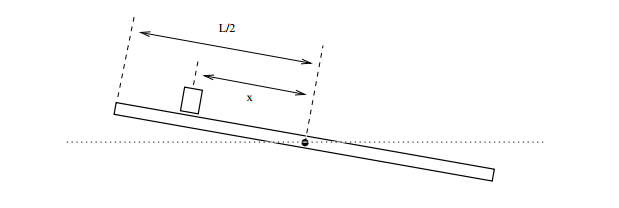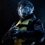# A really awesome situationSolving differential equations to solve physical problems is always fun, but it is never as fun as the situation i am going to present in this note,

Think about the situation shown in the figure, a tiny block is moving upon and along a rod that is hinged at the middle and can rotate in the vertical plane only,

Firstly you will observe that the rod rotates because the blocks weight has a net torque about it (when it is not at centre)

Let the distance along the rod be measured as 'x' and the angle the rod makes with the horizontal is 'a'

Qualitatively here are the Three situations you will expect would happen

1) The block moves too fast (at t=0) and so it slides off the rod very soon

2) The block moves too slow and comes to rest at the middle (ofcourse this can never practically happen, imagine the limiting case)

3) The block exactly reaches the centre when the rod becomes horizontal and both also reach the extremum at the same time, which means the system will be in oscillatory motion forever

Ok now let us see what The Math of the situation says,,

First let me make some assumptions so the differential equation we have to solve comes out to be simple

namely

1) The angle the rod rotates is very small, and thus this also tells that the systems initial energy is very small and hence we can neglect coriolis and centripetal forces in rotating frame (or their accelerations in ground frame)

2) All motions very slow

While the assumptions greatly limit us, i am forced to do so but the results are still awesome and Diverse

So, Let us analyse -

The torque on the rod is

$Nx\quad =\quad I\ddot { a }$

(worry not the directions and signs, they all depend on initial conditions and we can use negative numbers)

(also 'a' is the angle)

Now, for small angles, the normal reaction is roughly the weight of the block itself,

hence

$Nx\quad =\quad I\ddot { a } =mgx$

Now the force on the block along the rod assuming no friction is

$mgsin(a)-m{ w }^{ 2 }x=m\ddot { x } \simeq \quad mga$

Now to solve the two equations, i differentiate second one twice and substitute to get

$\\ m{ g }^{ 2 }x\quad =\quad I\ddddot { x } \\ \\ m{ g }^{ 2 }a\quad =\quad I\ddddot { a }$

Solving them yields me

$x\quad =\quad A{ e }^{ wt }+B{ e }^{ wt }+Csin(wt+D)\\ \\ a\quad =\quad A{ e }^{ wt }+B{ e }^{ wt }+Csin(wt+D)$

where $w=\left( \frac { m{ g }^{ 2 } }{ I } \right) ^{ \frac { 1 }{ 4 } }$

Which tell me all the information i need,

Now observe, if A and B are 0 as per initial conditions,

, Then the rod executes simple harmonic motion along with the block ,

If C and D and B are 0, then Quickly the block acquires enormous speed and distance and the block flies off or slides off (technically this is wrong as under my approximation this is impossible)

and motion dies off if A=C=D=0 as it exponentially decays

and there are several other cases, by setting some initial conditions, you can easily check it.Note by Mvs Saketh
6 years, 6 months ago

This discussion board is a place to discuss our Daily Challenges and the math and science related to those challenges. Explanations are more than just a solution — they should explain the steps and thinking strategies that you used to obtain the solution. Comments should further the discussion of math and science.

When posting on Brilliant:

• Use the emojis to react to an explanation, whether you're congratulating a job well done , or just really confused .
• Ask specific questions about the challenge or the steps in somebody's explanation. Well-posed questions can add a lot to the discussion, but posting "I don't understand!" doesn't help anyone.
• Try to contribute something new to the discussion, whether it is an extension, generalization or other idea related to the challenge.

MarkdownAppears as
*italics* or _italics_ italics
**bold** or __bold__ bold
- bulleted- list
• bulleted
• list
1. numbered2. list
1. numbered
2. list
Note: you must add a full line of space before and after lists for them to show up correctly
paragraph 1paragraph 2

paragraph 1

paragraph 2

[example link](https://brilliant.org)example link
> This is a quote
This is a quote
    # I indented these lines
# 4 spaces, and now they show
# up as a code block.

print "hello world"
# I indented these lines
# 4 spaces, and now they show
# up as a code block.

print "hello world"
MathAppears as
Remember to wrap math in $$ ... $$ or $ ... $ to ensure proper formatting.
2 \times 3 $2 \times 3$
2^{34} $2^{34}$
a_{i-1} $a_{i-1}$
\frac{2}{3} $\frac{2}{3}$
\sqrt{2} $\sqrt{2}$
\sum_{i=1}^3 $\sum_{i=1}^3$
\sin \theta $\sin \theta$
\boxed{123} $\boxed{123}$

Sort by:

This Interesting Situation , And I want to analyse it ! And Then Join the Discussion !

- 6 years, 6 months ago

yes please analyse it, and do try to solve it without approximation, which seems too complex for me,,

- 6 years, 6 months ago

I thought exactly the same situation some months ago and wanted to make a question on it but can't because of the so complex differential equation, but you did good job with the approximations, I didn't even thought about making approximations and then analysing the situation. @Mvs Saketh

- 6 years, 6 months ago

making approximations seems reasonable to me as far as the question will be about SHM, cause we dont expect SHM beyond the "slightly displaced" zone anyway

- 6 years, 6 months ago

I gave up, I make all Possible efforts But Fails every time! It is Too too complex for me too ! Your Approximations are great! Now I feel that how Little I know about "how to solve an diff. equation "!

- 6 years, 6 months ago

Actually i saw a similar question in the US physics team webpage, where the same question was posted except we were to assume that they are performing SHM and find the condition, and even there approximations were allowed however as we could directly assume SHM so one doesnt have to solve any differential equation,, but then when i analysed the differential equation , all these extra terms showing different kinds of motion than just SHM turned up But yes as you said actual differential equation is way too complex.

- 6 years, 6 months ago

agreed !

- 6 years, 6 months ago

This is a past USAPhO problem. (a variant, the general case instead of small oscillations)

- 1 year, 4 months ago